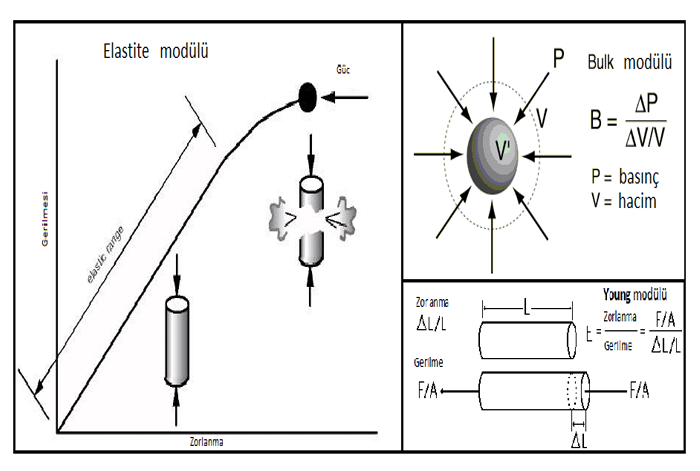## Elasticity TestsThe modulus of elasticity (or Young's Modulus) is a measure of the rate of stress change as a function of stress. Represents the slope of the straight line portion of a stress-strain curve.
This test method is used to determine the behavior of a sample under an axial tensile load. Common tensile test results include elastic limit, tensile strength, yield point, yield strength, elongation and Young's Modulus. Young's Module, usually N / mm2 (lbs / in2), is reported as MPA (psi).

The modulus of elasticity is somewhat lower than the control concrete made with the same weight / cm ratio of HVNP concrete made with a zeolite natural pozzolan (Uzal 2007). However, it is known that the elastic modulus of HVFA concrete is higher than Portland cement concrete, which has comparable strength (Malhotra and Ramezanianpour, 1994). This difference between HVFA and HVNP concrete mixes may be related to differences in the microstructure of the interfacial transition zone, but requires further study. In both types of high volume pozzolan concrete, the modulus of elasticity at 28 is close to 30 GPa or higher. days and therefore they are suitable for structural applications.
The Elasticity Module or 'Young Modulus' is a measure of the rate of change of strain as a function of stress. Regarding the tensile test, it can be called the Tensile Module. Represents the slope of the linear portion of a stress-strain curve.
A tensile test, also known as melt test, is probably the most basic type of mechanical test you can do on the material. Tensile tests are simple, relatively inexpensive and fully standardized. By pulling things, you will quickly determine how the material will react to the forces applied to the tension. As the material is drawn, you will see how long it will extend its strength.
This test method is used to determine the behavior of a sample under an axial tensile load. Common tensile test results include elastic limit, tensile strength, yield point, yield strength, elongation and Young's Modulus. Young's Module, usually N / mm2 (lbs / in2), is reported as MPA (psi).
A hard material, such as a diamond, has a high Young modulus and changes its shape slightly under an elastic load. A resilient material, such as rubber, has a low Young modulus and significantly changes its shape. A rigid material requires high loads to elastically deform - it should not be confused with a strong material, which requires high loads to constantly deform or break. The hardness of a component means how much it deviates under a given load. This depends on Young's modulus of material, as well as how the load (tension or bending) and the shape and size of the component. The specific stiffness is the division of the Young's modulus by density (but more accurately it should be called "specific modulus").
Hardness is only important in the design of products that can be bent by a certain amount (eg bridges, bicycles, furniture). Stiffness is important for springs that store elastic energy (eg jumpers, bungee ropes). For transport applications (eg airplanes, racing bikes) a minimum weight of stiffness is required. In these cases, materials with great hardness are best.
You can work with our laboratory EUROLAB for elasticity module tests.